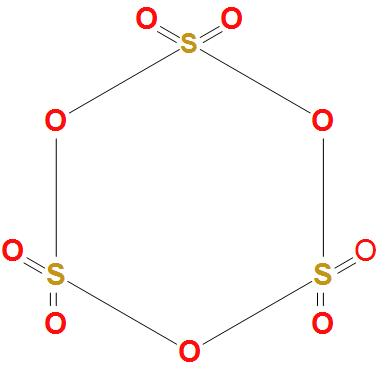# The number of S-S bond in sulphur trioxide trimer is:A. 3B. 2 C. 1D. 0Verified
175.2k+ views
Hint: As we know that sulphur trioxide trimer has the chemical formula $\left( {{S}_{3}}{{O}_{9}} \right)$. We can say it has nine oxygen atoms and three sulphur atoms. It is found that the chemical formula of sulphur trioxide is $S{{O}_{3}}$

- First of all let’s draw the structure of sulphur trioxide trimer $\left( {{S}_{3}}{{O}_{9}} \right)$-- We can see in the above structure of sulphur trioxide trimer $\left( {{S}_{3}}{{O}_{9}} \right)$ that; all the three sulphur atoms are bonded to the oxygen atoms on all the sides. It is clear that there is no bond formation taking place in between the sulphur atoms.
Hence, we can conclude that the correct option is (d), that is the number of S-S bond in sulphur trioxide trimer $\left( {{S}_{3}}{{O}_{9}} \right)$ is 0.